# Drawing functions in R with the curve function

## The `curve` function

In base R it is possible to draw a function with `curve`. By default the function will be plotted between 0 and 1 but you can change this range with `from` and `to`.

``````# Function
fun <- function(x) {
sin(sqrt(x ^ 2))
}

# Plot
curve(fun, from = -7, to = 7)``````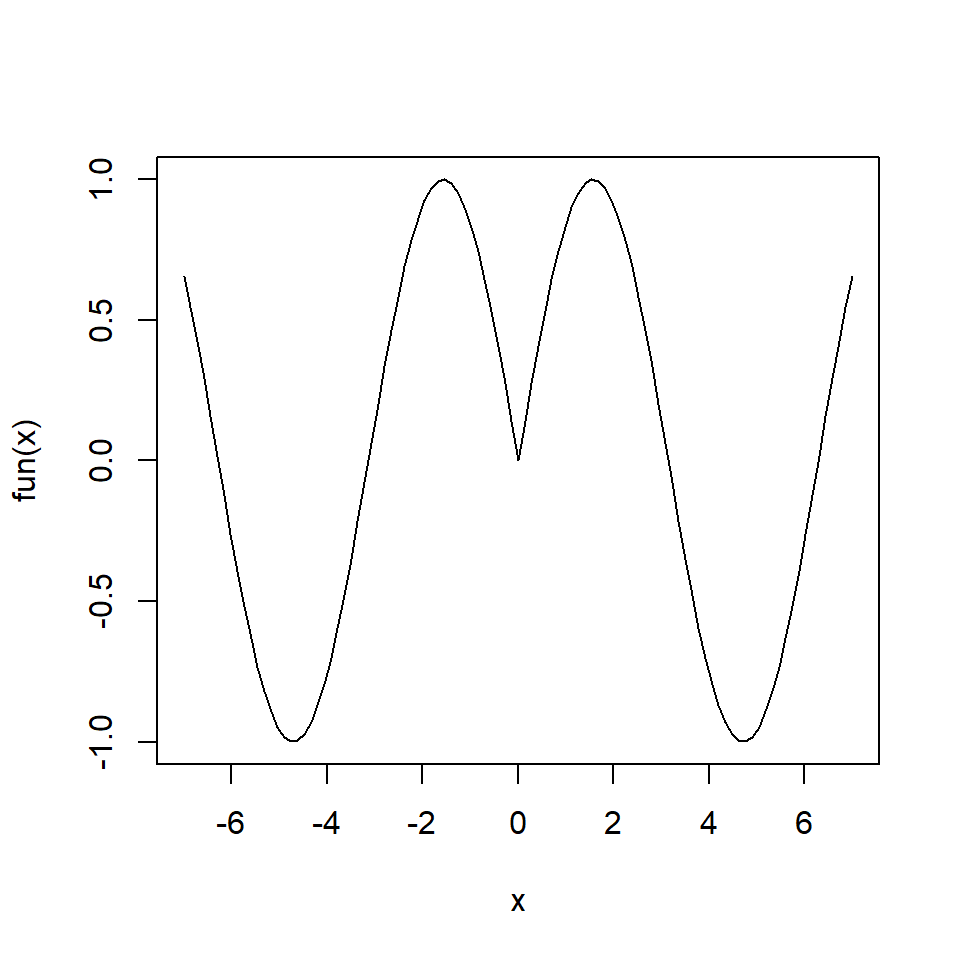Note that `curve` function is very useful for plotting functions such as `dnorm`, `dexp`, `cos`, `sin`, among others.

## Curve customization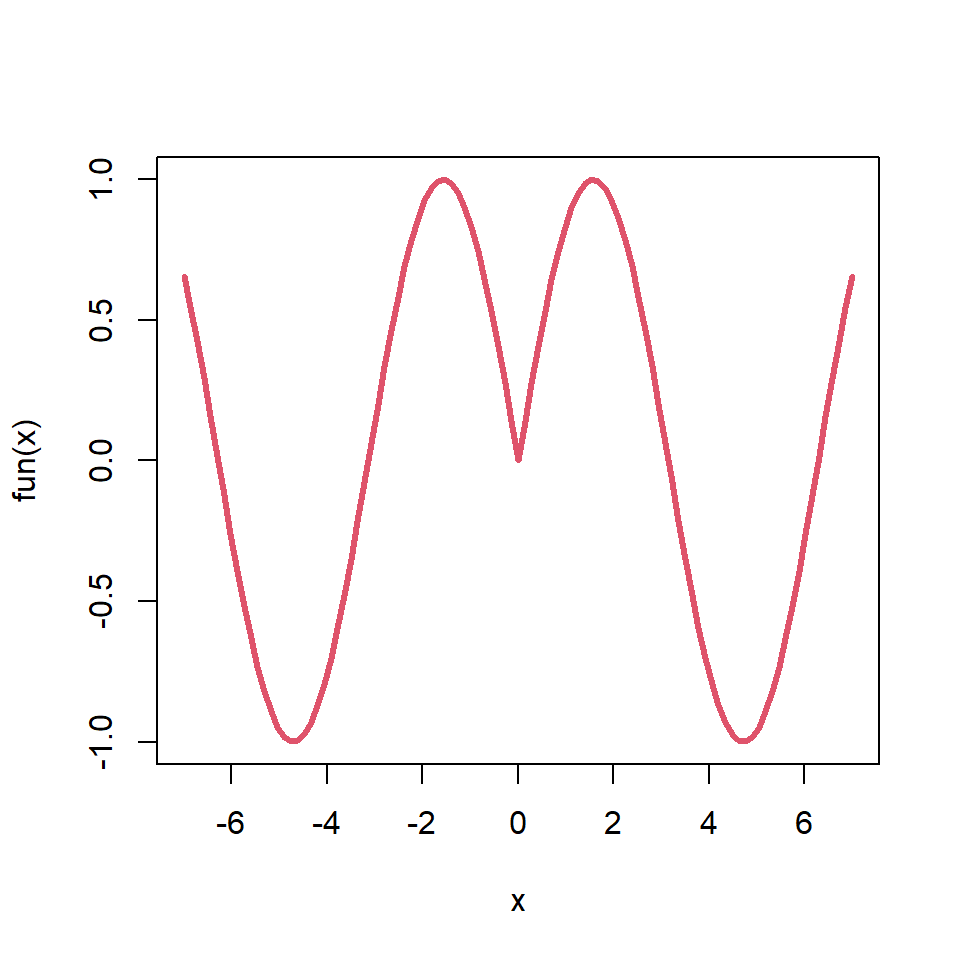The output of the `curve` function can be customized the same way as other base R plots. You can change the color, the line width or the line type, among other customizations.

``````# Function
fun <- function(x) {
sin(sqrt(x ^ 2))
}

# Plot
curve(fun, from = -7, to = 7,
col = 2, lwd = 3)``````

## Curve types

There are several line types available, which can be selected with `type` argument. In the following blocks of code we show a pair of examples.

Line and points

``````# Function
fun <- function(x) {
sin(sqrt(x ^ 2))
}

# Plot
curve(fun, from = -7, to = 7,
type = "o")``````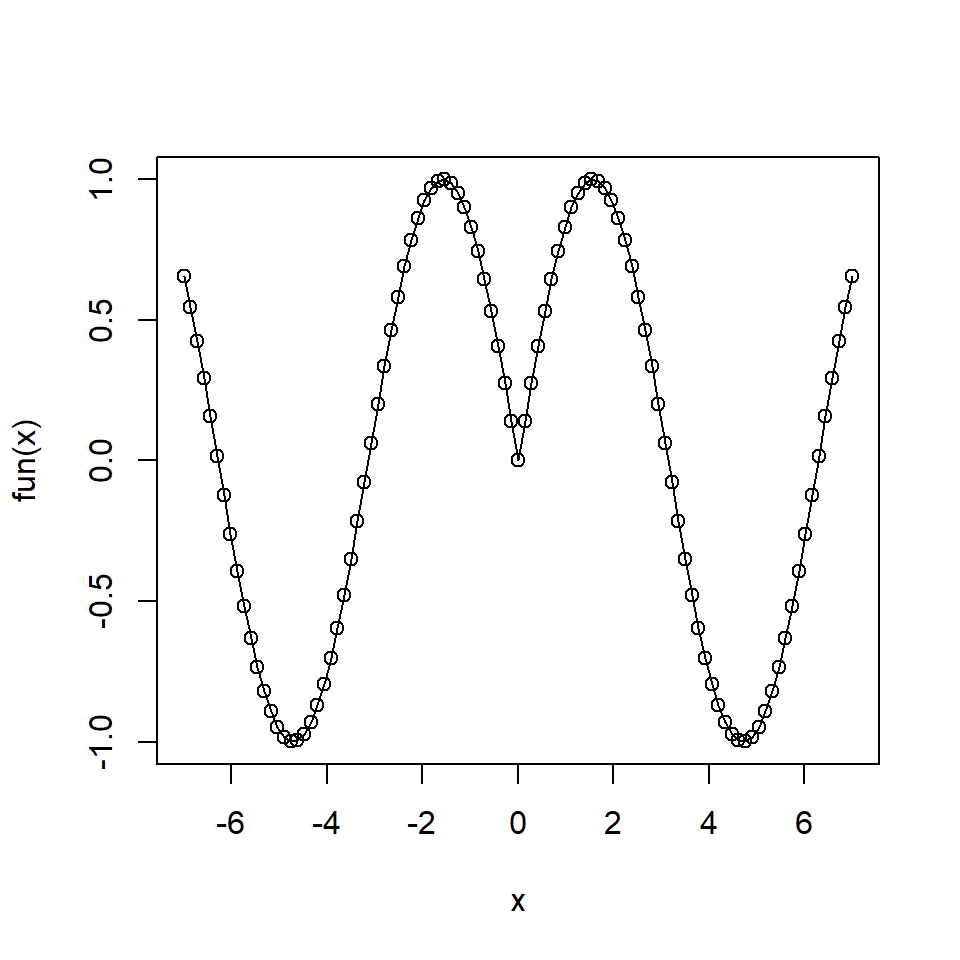Stairs plot

``````# Function
fun <- function(x) {
sin(sqrt(x ^ 2))
}

# Plot
curve(fun, from = -7, to = 7,
type = "s")``````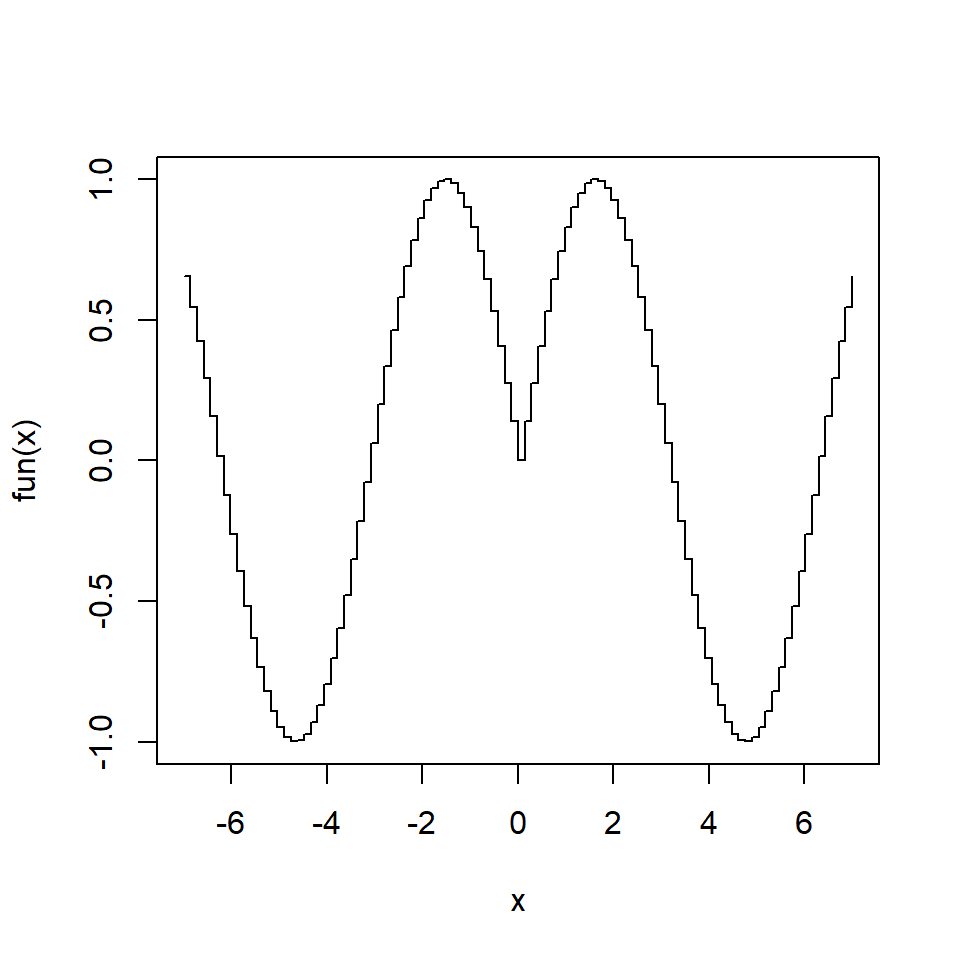Histogram-like

``````# Function
fun <- function(x) {
sin(sqrt(x ^ 2))
}

# Plot
curve(fun, from = -7, to = 7,
type = "h")``````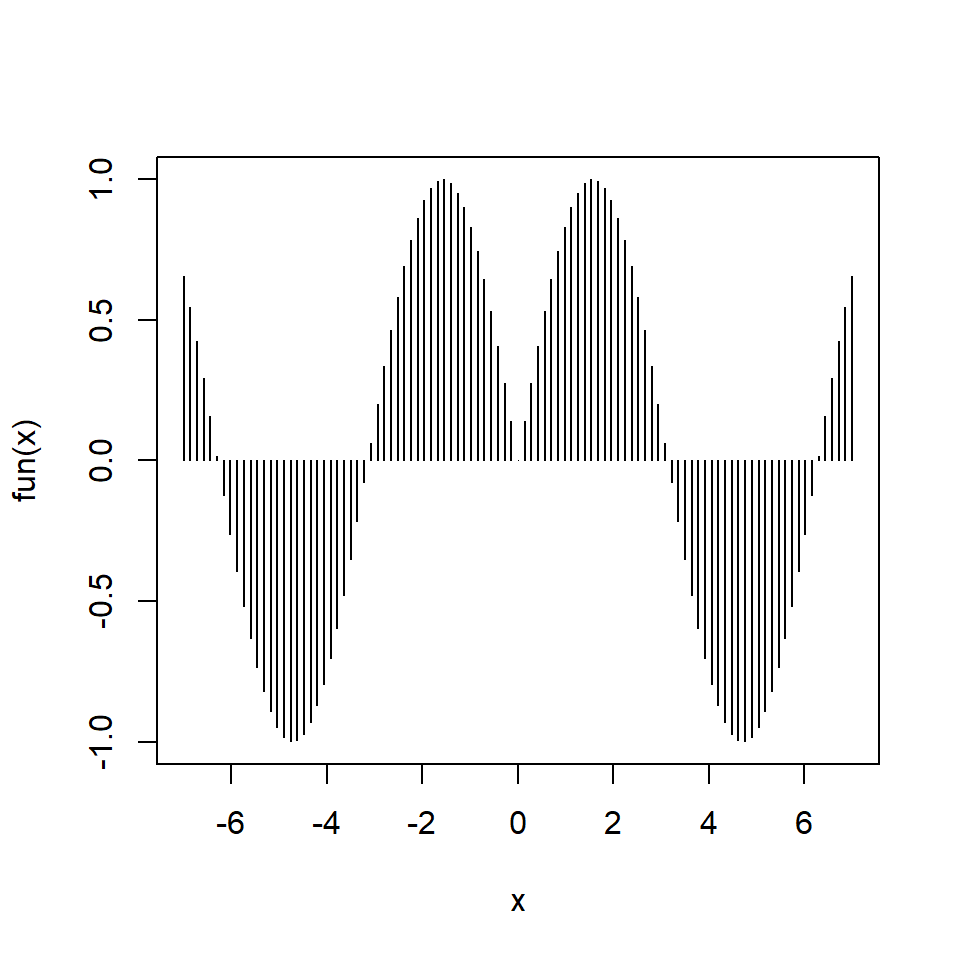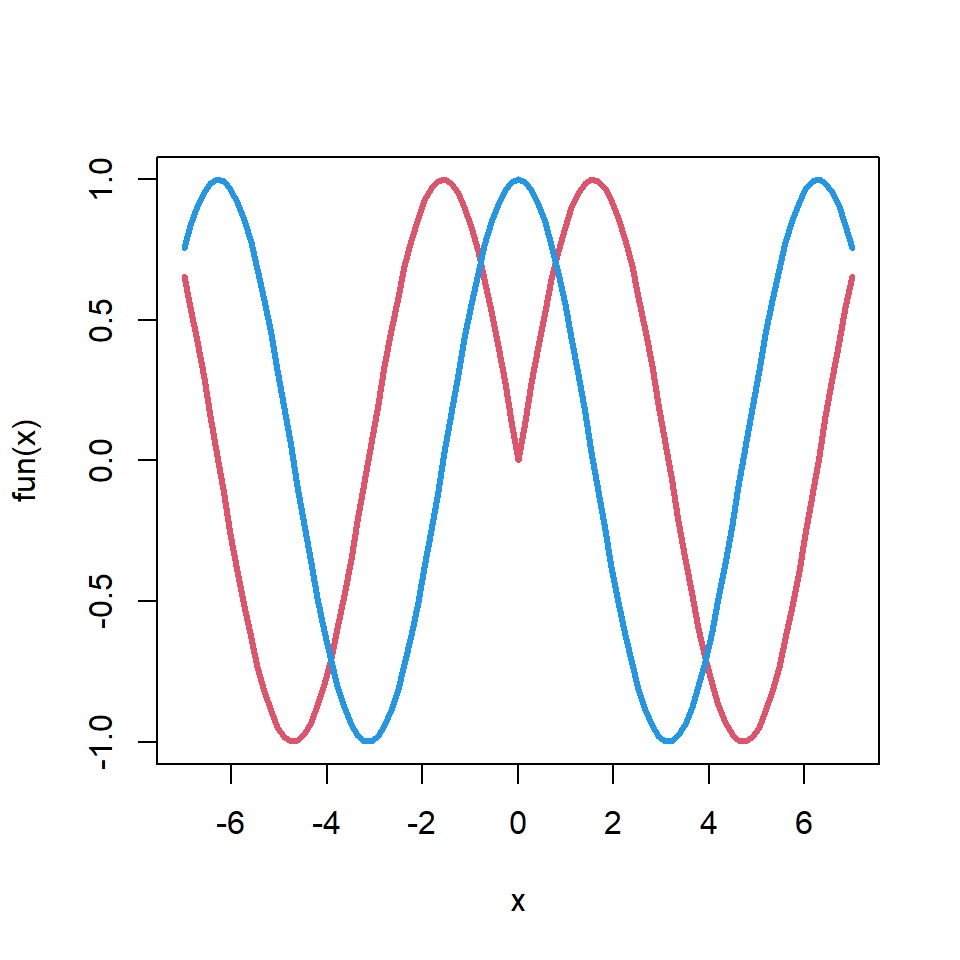You can also add multiple curves on the same plot setting `add = TRUE` on the second and posterior functions.

``````# Function
fun <- function(x) {
sin(sqrt(x ^ 2))
}

# Plot
curve(fun, from = -7, to = 7,
col = 2, lwd = 3)
curve(cos, col = 4, lwd = 3,